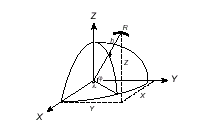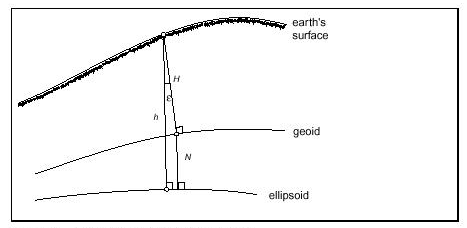`The Cartesian coordinate frame of reference used in GPS/GLONASS is called`
`Earth-Centered, Earth-Fixed (ECEF). ECEF uses three-dimensional XYZ coordinates`
`(in meters) to describe the location of a GPS user or satellite. The term "Earth-`
`Centered" comes from the fact that the origin of the axis (0,0,0) is located at the mass`
`center of gravity (determined through years of tracking satellite trajectories). The term`
`"Earth-Fixed" implies that the axes are fixed with respect to the earth (that is, they`
`rotate with the earth). The Z-axis pierces the North Pole, and the XY-axis defines the`
`equatorial plane (Figure 1).``Figure 1 ECEF Coordinate Reference Frame`
`ECEF coordinates are expressed in a reference system that is related to mapping`
`representations. Because the earth has a complex shape, a simple, yet accurate,`
`method to approximate the earth's shape is required. The use of a reference ellipsoid`
`allows for the conversion of the ECEF coordinates to the more commonly used`
`geodetic-mapping coordinates of Latitude, Longitude, and Altitude (LLA). Geodetic`
`coordinates can then be converted to a second map reference known as Mercator`
`Projections, where smaller regions are projected onto a flat mapping surface (that is,`
`Universal Transverse Mercator ­ UTM or the USGS Grid system).`
`A reference ellipsoid can be described by a series of parameters that define its`
`shape and which include a semi-major axis (a), a semi-minor axis (b) and its first`
`eccentricity (e 2) and its second eccentricity (e '2) as shown in Figure 2. Depending on`
`the formulation used, ellipsoid "flattening" may be required.``    Figure 2 Ellipsoid Parameters`
`For global applications, the geodetic reference (datum) used for GPS is the World`
`Geodetic System 1984 (WGS84). This ellipsoid has its origin coincident with the`
`ECEF origin. The X-axis pierces the Greenwich meridian (where longitude = 0`
`degrees) and the XY plane make up the equatorial plane (latitude = 0 degrees).`
`Altitude is described as the perpendicular distance above the ellipsoid surface (which`
`not to be confused with the mean sea level datum).`
`Figure 3 ECEF and Reference Ellipsoid`
`LLA to ECEF`
`The conversion between the two reference coordinate systems can be performed`
`using closed formulas (although iteration methods also exist). The conversion from`
`LLA to ECEF (in meters) is shown below.`
`X = [N + H] cos ¢. cos £                    (1)`
`Y = [N + H] cos ¢. sin £                    (2)`
`Z = [(b 2 /a 2) N + h] sin ¢.                  (3)`
`Where:`
`¢ = latitude`
`£= longitude`
`h = height above ellipsoid (meters)`
`N = Prime Vertical of Curvature (meters) is defined as:`
`                a 2`
`N = ____________________                            (4)`
`        [a 2 cos 2 ¢. + b 2 sin 2 £ ] 1/2`
`ECEF to LLA`
`    The conversion between XYZ and LLA is slightly more involved but can be`
`achieved using the following closed formula set:``¢ = arctan [ _________________                ]      (5)`
`£ = arctan    [ ____    ]                            (6)`
`                        X`
`h = [  P`
`_______      ] ­ N                              (7)`
`        Cos ¢`
`Where auxiliary values are:`
`¥ = arctan [ _____      ]                              (8)`
`                    p b`
`p = [X 2 +Y 2 ] 1/2                                                      (9)`
`GPS Heights`
`The height determined by GPS measurements relates to the perpendicular distance`
`above the reference ellipsoid and should not be confused with the more well-known`
`height datum Mean Sea Level (MSL). The datum that defines the MSL (also called`
`the geoid) is a complex surface that requires dense and accurate gravity data to define`
`its shape. The WGS84 ellipsoid approximates the geoid on a worldwide basis with`
`deviations between the two datums never exceeding 100 meters. The transformation`
`between the two surfaces is illustrated in Figure 4.`
`Figure 4 Ellipsoid and MSL Reference Datums`
`p ­ e 2 a cos 3 ¥`
`Z + e '2 b sin 3 ¥`
`Y`
`Z a``     `
`The conversion between the two reference datums is shown by:`
`h = H + N                                                (10)`
`where:`
`h        ellipsoidal height (Geodetic)`
`H      orthometric height (MSL)`
`N      geoid separation (undulation)`
`Note ­ The ellipsoid/geoid separation ranges from a value of +100 meters to`
`­100 meters.`
`Although the conversion between the different height datums is straightforward, the`
`accuracy at which the undulation is known (N) varies greatly with gravity`
`measurement data density. It is even more difficult to determine in mountainous`
`regions where mass distribution can vary rapidly.`